# 模式搜索的暴力方法

### 关于暴力及其与神经网络的区别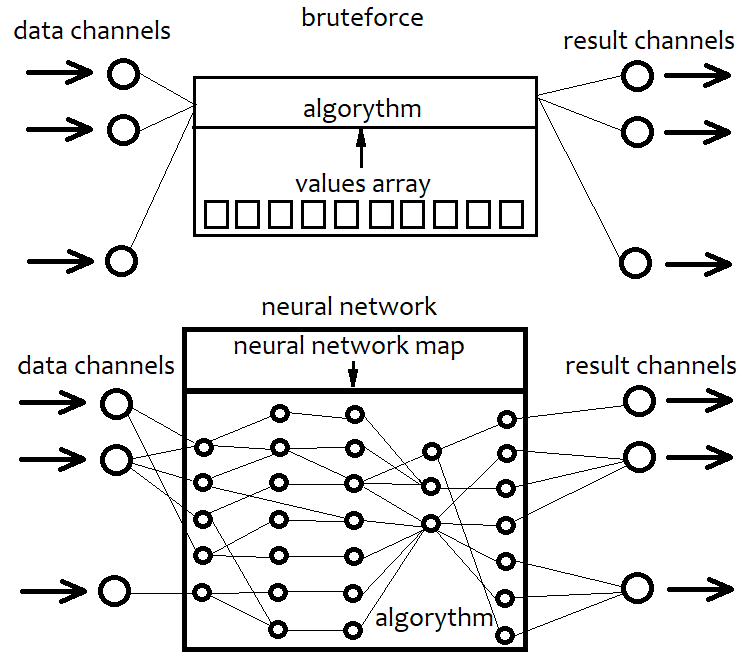### 我的暴力与优化算法

Y = Cs+Cs*(x-x0)^1 + Cs*(x-x0) ^2 + ... + Cs[i]*(x-x0)^n

Y = C+C*x^1 + C*x^2 + ... + C[i]*x^n + ...= Sum(0,+infinity)(C[i]x^i)

Y = Sum(0,+Infinity)( C[i]*Product variant(x1^p1*x2^p2...*xN^pN)

### 为任务构建简单模板

```double C1[] = { %%%CVALUES%%% };//array of coefficients
int CNum=%%%CNUMVALUE%%%;//number of candlesticks in the formula
int DeepBruteX=%%%DEEPVALUE%%%;//formula depth
int DatetimeStart=%%%DATETIMESTART%%%;//start point in time
```

```int NumCAll=0;//size of the array of coefficients
void DeepN(int Nums,int deepC=1)//intermediate fractal
{
for ( int i=0; i<Nums; i++ )
{
if (deepC > 1)
{
DeepN(Nums,deepC-1);
}
else
{
NumCAll++;
}
}
}

void CalcDeepN(int Nums,int deepC=1)//to launch calculations
{
NumCAll=0;
for ( int i=0; i<deepC; i++ )
{
DeepN(Nums,i+1);
}
}
```

```double ValW;//the number where everything is multiplied (and then added to ValStart)
uint NumC;//the current number for the coefficient
double ValStart;//the number where to add everything
void Deep(double &Ci0[],int Nums,int deepC=1,double Val0=1.0)//calculate the sum of one degree
{
for ( int i=0; i<Nums; i++ )
{
if (deepC > 1)
{
ValW=(Close[i+1]-Open[i+1])*Val0;
Deep(Ci0,Nums,deepC-1,ValW);
}
else
{
ValStart+=Ci0[NumC]*(Close[i+1]-Open[i+1])*Val0/Point;
NumC++;
}
}
}

void CalcDeep(double &Ci0[],int Nums,int deepC=1)//calculate the entire polynomial
{
NumC=0;
ValStart=0.0;
for ( int i=0; i<deepC; i++ )
{
Deep(Ci0,Nums,i+1);
}
}
```

```double Val;
{
Val=0;
if ( DeepBruteX <= 1 )
{
for ( int i=0; i<ArraySize(C1); i++ )
{
Val+=C1[i]*(Close[i+1]-Open[i+1])/Point;
}
return Val;
}
else
{
CalcDeep(C1,CNum,DeepBruteX);
return ValStart;
}
}
```

```void Trade()
{
double Value;

if ( Value > ValueCloseE)
{
if ( !bInvert )
{
}
else
{
CloseSellF();
}
}

if ( Value < -ValueCloseE)
{
if ( !bInvert )
{
CloseSellF();
}
else
{
}
}

if ( double(TimeCurrent()-DatetimeStart)/86400.0 <= DaysToTrade && Value > ValueOpenE && Value <= ValueOpenEMax )
{
if ( !bInvert ) SellF();
}

if ( double(TimeCurrent()-DatetimeStart)/86400.0 <= DaysToTrade && Value < -ValueOpenE && Value >= -ValueOpenEMax )
{
else SellF();
}

}
```

```void CalcTimer()
{
if ( Time > PrevTimeAlpha )
{
if ( PrevTimeAlpha > 0 )
{
}
PrevTimeAlpha=Time;
}
}
```

```   void GenerateC()
{
double RX;
if ( DeepBrute > 1 ) CalcDeepN(CandlesE,DeepBrute);
else NumCAll=CandlesE;
for ( int j=0; j<VariantsE; j++ )
{
ArrayResize(Variants[j].Ci,NumCAll,0);
Variants[j].CNum=CandlesE;
Variants[j].ANum=NumCAll;
Variants[j].DeepBruteX=DeepBrute;
RX=MathRand()/32767.0;
for ( int i=0; i<Variants[j].ANum; i++ )
{
if ( RE == RANDOM_TYPE_1 ) Variants[j].Ci[i]=double(MathRand())/32767.0;
if ( RE == RANDOM_TYPE_2 )
{
if ( MathRand()/32767.0 >= 0.5  )
{
Variants[j].Ci[i]=double(MathRand())/32767.0;
}
else
{
Variants[j].Ci[i]=double(-MathRand())/32767.0;
}
}
if ( RE == RANDOM_TYPE_3 )
{
if ( MathRand()/32767.0 >= RX  )
{
if ( MathRand()/32767.0 >= RX+(1.0-RX)/2.0  )
{
Variants[j].Ci[i]=double(MathRand())/32767.0;
///Print(Variants[j].Ci[i]);
}
else
{
Variants[j].Ci[i]=double(-MathRand())/32767.0;
}
}
else
{
Variants[j].Ci[i]=0.0;
}
}
if ( RE == RANDOM_TYPE_4 )
{
if ( MathRand()/32767.0 >= RX  )
{
Variants[j].Ci[i]=double(MathRand())/32767.0;
}
else
{
Variants[j].Ci[i]=0.0;
}
}
}
}
}
```

MQL4和MQL5中的暴力算法原型可以在文章附件中找到。这里我不提供我的交易函数实现，因为目的只是展示如何在模板框架内实现该方法。如果您有兴趣查看整个实现，请查看附件。所有的EA交易和其他必要的材料都可以在本文的附件中找到。我的模板有很多多余的东西，包括不必要的函数或变量，可以通过某种方式进行优化。我个人不介意。如果有什么干扰操作，我就把它移走。对我来说更重要的是现在一切正常。我一直在开发一些东西，所以我没有时间把每一个细节都做到完美。我也认为没有理由将所有过程和变量存储在类中，而这可以提高代码顺序和可读性。模板非常简单。程序将使用的带引号的文件将由一个专门的EA交易生成，该EA根据历史运行，并将柱形图数据写入一个文本文件，该文件的结构便于程序读取。我不会提供这个EA的代码在这里，因为它可以很容易地开发。

### 使用程序查找和分析模式

• 第一个时间段:  2020.01.13 - 2020.02.16
• 第二个时间段:  2020.02.13 - 2020.03.15
• 第三个时间段:  2020.03.13 - 2020.04.18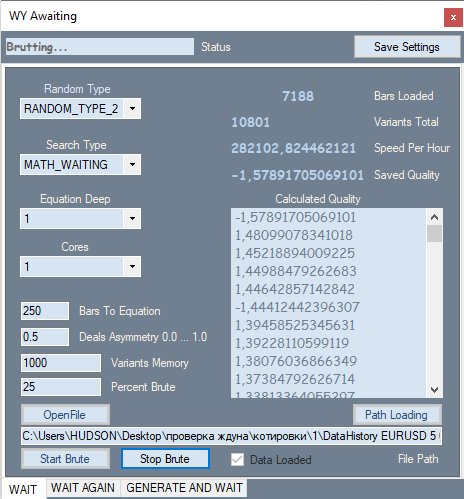P_Factor=(Profit-Loss)/(Profit+Loss).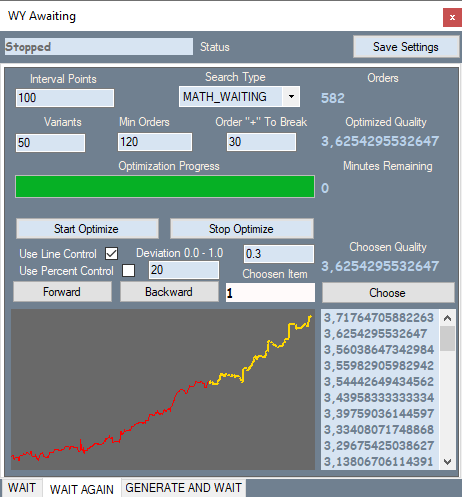Deviation = Max(|Profit[i]-LineProfit[i]|)/EndProfit.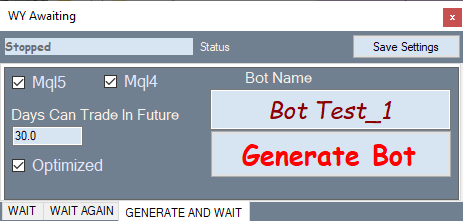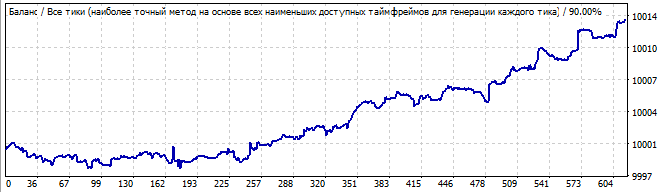未来10天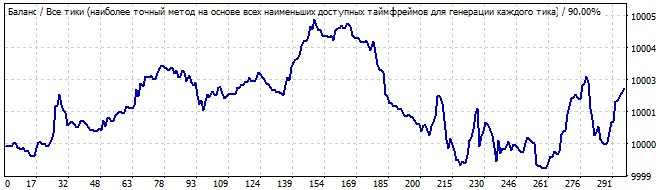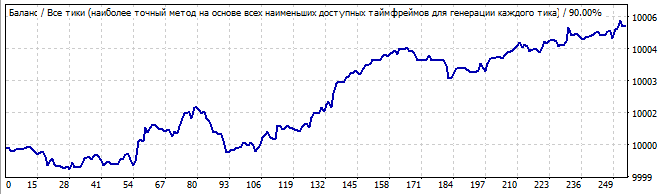未来10天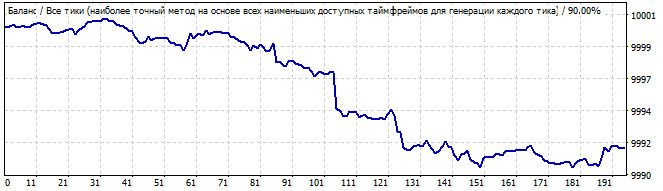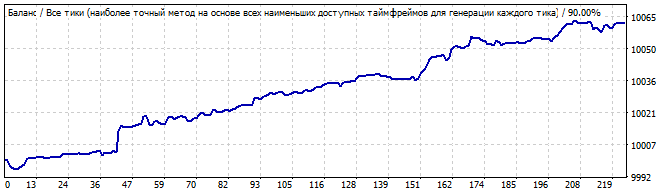未来10天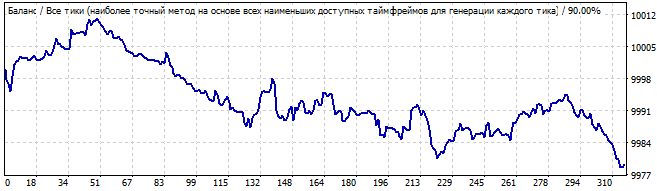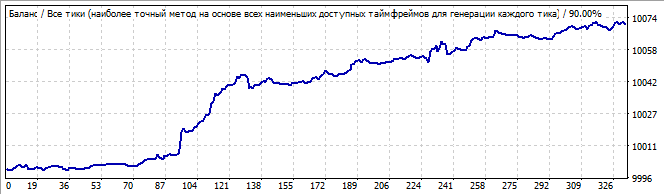未来10天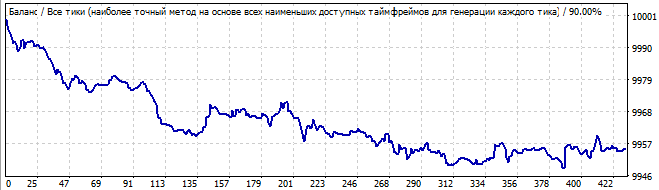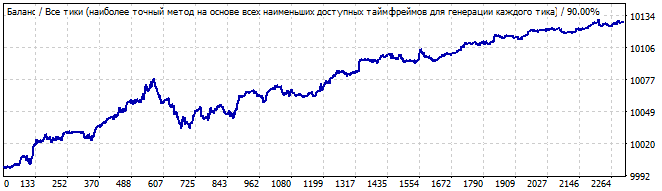未来10天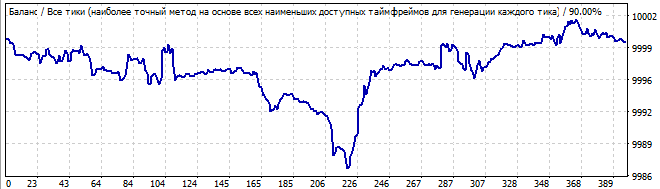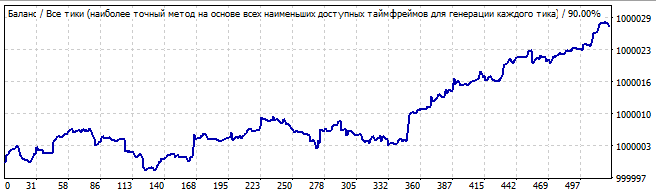未来10天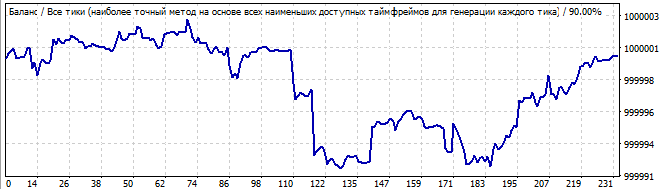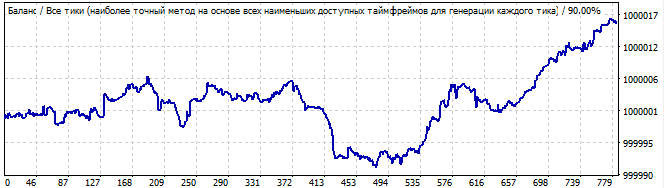未来10天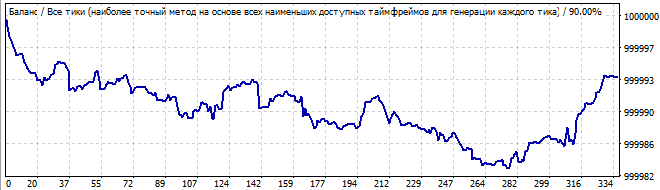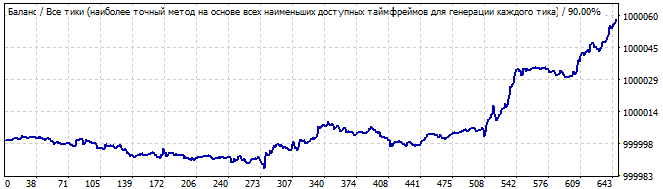未来10天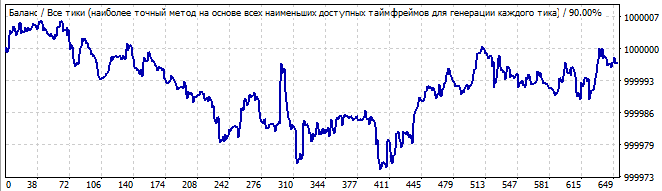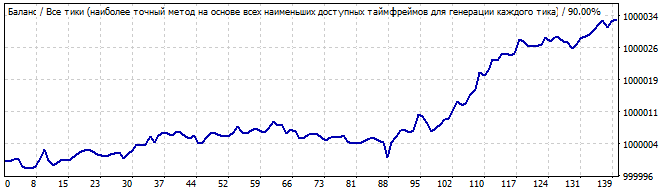未来10天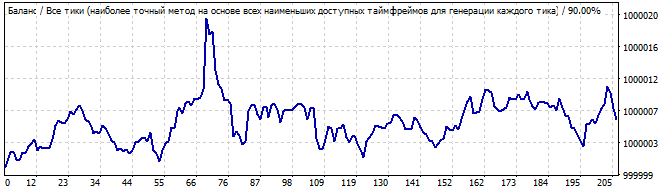暴力期间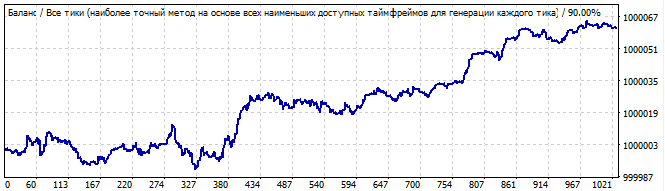未来10天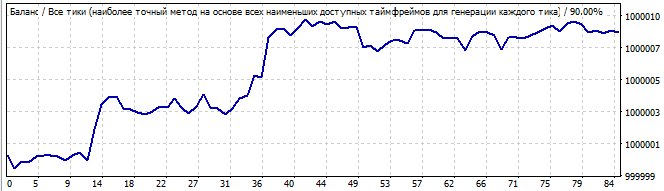暴力期间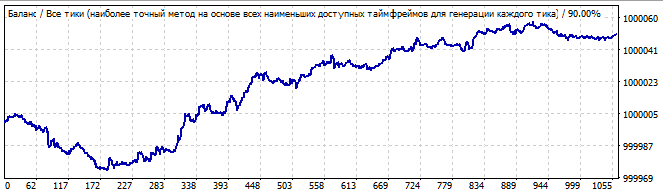未来10天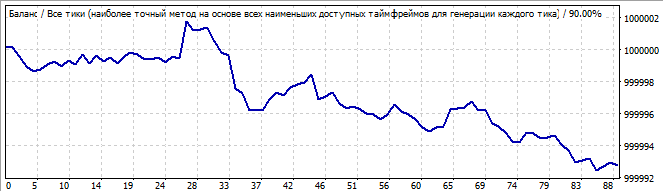### 我们研究的结论和数学原理

• 在以后的每个测试中，总有一个点是图表反转，然后公式反转。
• 反转可以平稳发生，也可以瞬间发生，但它总是存在的。
• 绝大多数图形通常在将来会下降，
• 有时，这种模式在开始时会持续一段时间。
• 未来的所有测试都表明，这种模式的工作方向是相反的。
• 如果余额曲线偏离了直线，那么延续的可能性就要低得多。
• 在发现的最好的测试中，这种模式持续工作了一两天。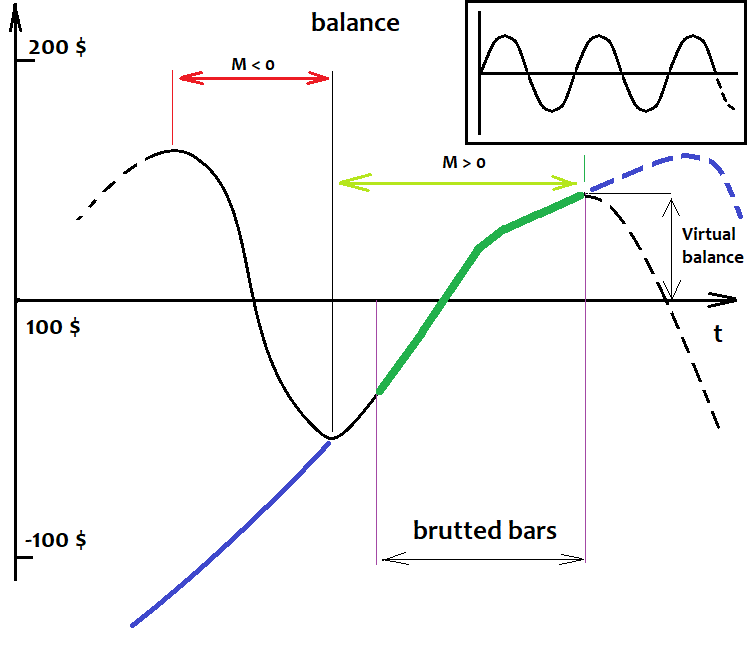### 如何确定波动发生的相对水平？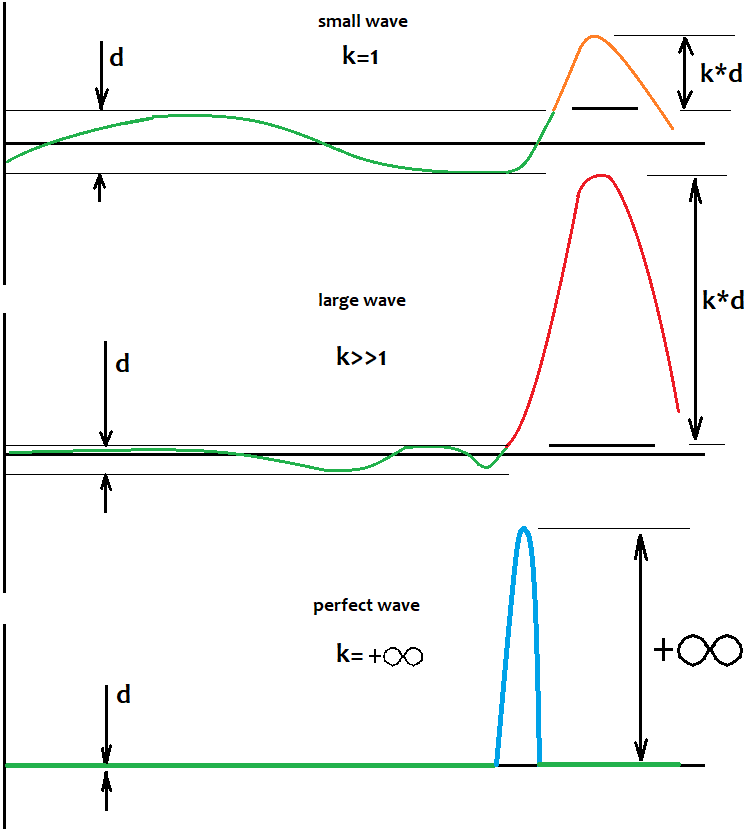### 为了完成这张图片，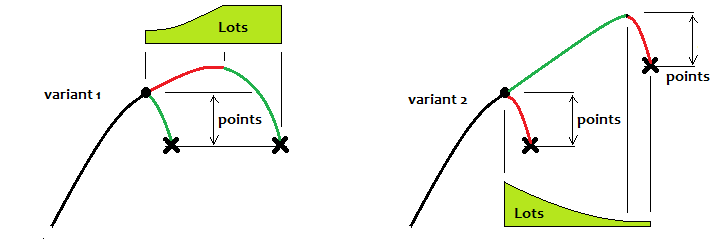## 量化课程

移动端课程